# wavedec2

2-D wavelet decomposition

## Syntax

``[C,S] = wavedec2(X,N,wname)``
``[C,S] = wavedec2(X,N,LoD,HiD)``

## Description

example

````[C,S] = wavedec2(X,N,wname)` returns the wavelet decomposition of the matrix `X` at level `N` using the wavelet `wname`. The output decomposition structure consists of the wavelet decomposition vector `C` and the bookkeeping matrix `S`, which contains the number of coefficients by level and orientation. NoteFor `gpuArray` inputs, the supported modes are `'symh'` (`'sym'`) and `'per'`. If the input is a `gpuArray`, the discrete wavelet transform extension mode used by `wavedec2` defaults to `'symh'` unless the current extension mode is `'per'`. See the example Multilevel 2-D Discrete Wavelet Transform on a GPU. ```
````[C,S] = wavedec2(X,N,LoD,HiD)` returns the wavelet decomposition using the specified lowpass and highpass decomposition filters `LoD` and `HiD`, respectively. See `wfilters` for details.```

## Examples

collapse all

This example shows how to extract and display images of wavelet decomposition level details.

Load an image. Perform a level 2 wavelet decomposition of the image using the `haar` wavelet.

```load woman [c,s]=wavedec2(X,2,'haar');```

Extract the level 1 approximation and detail coefficients.

```[H1,V1,D1] = detcoef2('all',c,s,1); A1 = appcoef2(c,s,'haar',1);```

Use `wcodemat` to rescale the coefficients based on their absolute values. Display the rescaled coefficients.

```V1img = wcodemat(V1,255,'mat',1); H1img = wcodemat(H1,255,'mat',1); D1img = wcodemat(D1,255,'mat',1); A1img = wcodemat(A1,255,'mat',1); subplot(2,2,1) imagesc(A1img) colormap pink(255) title('Approximation Coef. of Level 1') subplot(2,2,2) imagesc(H1img) title('Horizontal Detail Coef. of Level 1') subplot(2,2,3) imagesc(V1img) title('Vertical Detail Coef. of Level 1') subplot(2,2,4) imagesc(D1img) title('Diagonal Detail Coef. of Level 1')```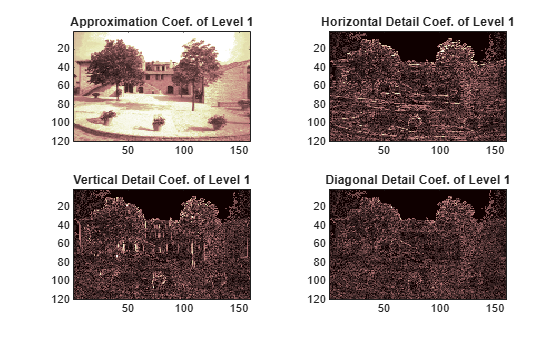Extract the level 2 approximation and detail coefficients.

```[H2,V2,D2] = detcoef2('all',c,s,2); A2 = appcoef2(c,s,'haar',2);```

Use `wcodemat` to rescale the coefficients based on their absolute values. Display the rescaled coefficients.

```V2img = wcodemat(V2,255,'mat',1); H2img = wcodemat(H2,255,'mat',1); D2img = wcodemat(D2,255,'mat',1); A2img = wcodemat(A2,255,'mat',1); figure subplot(2,2,1) imagesc(A2img) colormap pink(255) title('Approximation Coef. of Level 2') subplot(2,2,2) imagesc(H2img) title('Horizontal Detail Coef. of Level 2') subplot(2,2,3) imagesc(V2img) title('Vertical Detail Coef. of Level 2') subplot(2,2,4) imagesc(D2img) title('Diagonal Detail Coef. of Level 2')```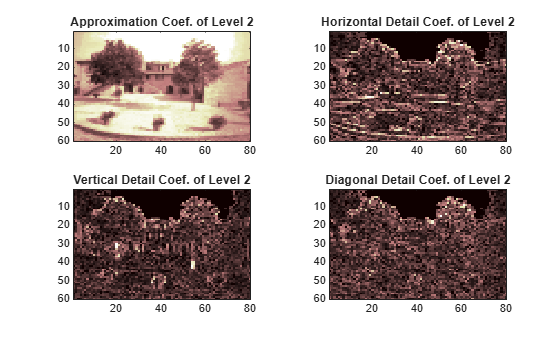This example shows the structure of `wavedec2` output matrices.

```load woman imagesc(X) colormap(map)```Save the current discrete wavelet transform extension mode.

`origMode = dwtmode('status','nodisplay');`

Change to periodic boundary handling. The `dwtmode` function displays a message indicating that the DWT extension mode is changing.

`dwtmode('per')`
``` !!!!!!!!!!!!!!!!!!!!!!!!!!!!!!!!!!!!!!!! ! WARNING: Change DWT Extension Mode ! !!!!!!!!!!!!!!!!!!!!!!!!!!!!!!!!!!!!!!!! ***************************************** ** DWT Extension Mode: Periodization ** ***************************************** ```

Perform a level 3 decomposition of the image using the `db1` (Haar) wavelet.

`[c,s] = wavedec2(X,3,'db1');`

Return the number of elements in the image `X` and coefficient vector `c`. Confirm the number of elements in each are equal.

`numel(X)`
```ans = 65536 ```
`numel(c)`
```ans = 65536 ```

Display the bookkeeping matrix `s`. The first row displays the dimensions of the coarse scale approximation of the image. The last row displays the dimensions of the original image. The intermediate rows display the dimensions of the detail coefficients at the three levels of the decomposition, proceeding from coarse to fine scale.

`s`
```s = 5×2 32 32 32 32 64 64 128 128 256 256 ```

Reset discrete wavelet transform extension mode to its original mode.

`dwtmode(origMode,'nodisplay')`

Refer to GPU Support by Release (Parallel Computing Toolbox) to see what GPUs are supported.

Load an image. Put the image on the GPU using `gpuArray`. Save the current extension mode.

```load mask imgg = gpuArray(X); origMode = dwtmode('status','nodisp');```

Use `dwtmode` to change the extension mode to zero-padding. Obtain the three-level DWT of the image on the GPU using the `db4` wavelet.

```dwtmode('zpd','nodisp') [c,s] = wavedec2(imgg,3,'db4');```

The current extension mode `zpd` is not supported for `gpuArray` input. Therefore, the DWT is instead performed using the `sym` extension mode. To confirm this, set the extension mode to `sym` and take DWT of `noisdoppg`, then compare with the previous result.

```dwtmode('sym','nodisp') [csym,ssym] = wavedec2(imgg,3,'db4'); [max(abs(c-csym)) max(abs(s-ssym))]```
```ans = 0 0 0 ```

Set the current extension mode to `per` and obtain the three-level DWT of `imgg`. The extension mode `per` is supported for `gpuArray` input. Confirm the result is different from the `sym` results.

```dwtmode('per','nodisp') [cper,sper] = wavedec2(imgg,3,'db4'); [length(csym) ; length(cper)]```
```ans = 2×1 71542 65536 ```
`ssym`
```ssym = 5×2 38 38 38 38 69 69 131 131 256 256 ```
`sper`
```sper = 5×2 32 32 32 32 64 64 128 128 256 256 ```

Restore the extension mode to the original setting.

`dwtmode(origMode,'nodisp')`

## Input Arguments

collapse all

Input data, specified as a real-valued M-by-N matrix representing an indexed image, or an M-by-N-by-3 array representing a truecolor image. For more information on truecolor images, see RGB (Truecolor) Images.

Data Types: `double`

Decomposition level, specified as a positive integer. `wavedec2` does not enforce a maximum level restriction. Use `wmaxlev` to determine the maximum decomposition level possible of the matrix `X` using the wavelet `wname`. The maximum level is the last level for which at least one coefficient is correct.

Data Types: `double`

Analyzing wavelet, specified as a character vector or string scalar.

Note

`wavedec2` supports only Type 1 (orthogonal) or Type 2 (biorthogonal) wavelets. See `wfilters` for a list of orthogonal and biorthogonal wavelets.

Wavelet decomposition filters associated with an orthogonal or biorthogonal wavelet, specified as even-length real-valued vectors. `LoD` is the lowpass decomposition filter, and `HiD` is the highpass decomposition filter. See `wfilters` for details.

Data Types: `double`

## Output Arguments

collapse all

Wavelet decomposition vector. The vector `C` contains the approximation and detail coefficients organized by level. The bookkeeping matrix `S` is used to parse `C`.

The vector `C` is organized as A(`N`), H(`N`), V(`N`), D(`N`), H(`N`-1), V(`N`-1), D(`N`-1), …, H(1), V(1), D(1), where A, H, V, and D are each a row vector. Each vector is the column-wise storage of a matrix.

• A contains the approximation coefficients.

• H contains the horizontal detail coefficients.

• V contains the vertical detail coefficients.

• D contains the diagonal detail coefficients.

Bookkeeping matrix. The matrix `S` contains the dimensions of the wavelet coefficients by level and is used to parse the wavelet decomposition vector `C`.

• `S(1,:)` = size of approximation coefficients(`N`).

• `S(i,:)` = size of detail coefficients(`N-i+2`) for `i` = 2, ...`N+1` and `S(N+2,:) = size(X)`.

The following diagram shows the relationship between `C` and `S` in the wavelet decomposition of a 512-by-512 matrix.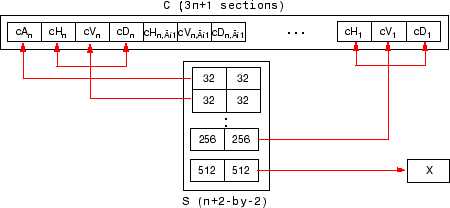When `X` represents an indexed image, the output arrays cA, cH, cV, and cD are m-by-n matrices. When `X` represents a truecolor image, it is an m-by-n-by-3 array, where each m-by-n matrix represents a red, green, or blue color plane concatenated along the third dimension. The size of vector `C` and the size of matrix `S` depend on the type of analyzed image.

For a truecolor image, the decomposition vector `C` and the corresponding bookkeeping matrix `S` can be represented as shown.## Algorithms

For images, an algorithm similar to the one-dimensional case is possible for two-dimensional wavelets and scaling functions obtained from one-dimensional vectors by tensor product. This kind of two-dimensional DWT leads to a decomposition of approximation coefficients at level j in four components: the approximation at level j+1 and the details in three orientations (horizontal, vertical, and diagonal).

The chart describes the basic decomposition step for images: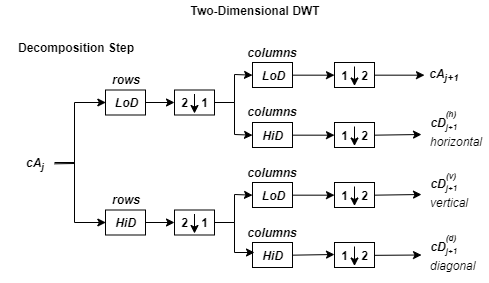where

•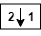— Downsample columns: keep the even-indexed columns.

•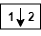— Downsample rows: keep the even-indexed rows.

•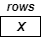— Convolve with filter X the rows of the entry.

•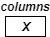— Convolve with filter X the columns of the entry.

and

Initialization: cA0 = s.

So, for J = 2, the two-dimensional wavelet tree has the form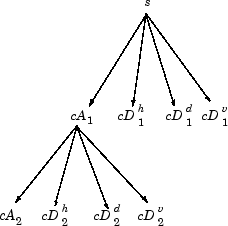Daubechies, Ingrid. Ten Lectures on Wavelets. CBMS-NSF Regional Conference Series in Applied Mathematics 61. Philadelphia, Pa: Society for Industrial and Applied Mathematics, 1992.

 Mallat, S.G. “A Theory for Multiresolution Signal Decomposition: The Wavelet Representation.” IEEE Transactions on Pattern Analysis and Machine Intelligence 11, no. 7 (July 1989): 674–93. https://doi.org/10.1109/34.192463.

 Meyer, Y. Wavelets and Operators. Translated by D. H. Salinger. Cambridge, UK: Cambridge University Press, 1995.